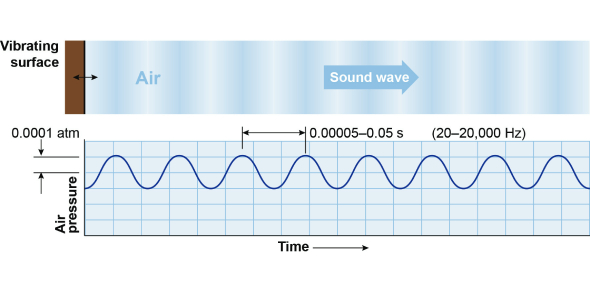# The Sound Waves Trivia Quiz!

15 Questions | Total Attempts: 58Settings.

• 1.
It is composed of waves of compression and refraction in with the human ear is sensitive.
• A.

Wave

• B.

Sound

• C.

Music

• D.

Energy

• 2.
It refers to how high or low a sound seems.
• A.

Patch

• B.

Sound

• C.

Pitch

• D.

Volume

• 3.
Sound waves carry _________
• A.

Longitudinal waves

• B.

Transverse wave

• C.

Energy

• D.

Motion

• 4.
When Mr. Sinyard yells his name in a cave, the sound reflects off the walls of the cave and travels back to his ears.
• A.

Refraction

• B.

Pitch

• C.

Amplitude

• D.

Echo

• 5.
It is an amount of sound energy that passes through a square meter of space in one second.
• A.

Intensity

• B.

Reverberation

• C.

Pitch

• D.

Transmission

• 6.
It is the material that sound waves can travel. It can travel through solid, liquid, and gas but not in a vacuum.
• A.

Waves

• B.

Door

• C.

Water

• D.

Meduim

• 7.
What unit is used to measure frequency?
• A.

Joule

• B.

Gram

• C.

Hertz

• D.

Newton

• 8.
It refers to inducing vibration of natural rate source having the same frequency.
• A.

Resonance

• B.

Reflection

• C.

Refraction

• D.

Rarefraction

• 9.
When the sound waves bend or diffract around corners or barriers like doors and walls. It is called?
• A.

Refraction of sound

• B.

Diffraction of sound

• C.

Reflection of sound

• D.

Speed of sound

• 10.
It is a tone quality and used to distinguished two different sounds that have the same pitch and loudness.
• A.

Density

• B.

Rhythm

• C.

Timbre

• D.

Elasticity

• 11.
In the characteristics of musical sound, it has a basic element of music.
• A.

Note

• B.

Intensity

• C.

Pitch

• D.

Harmony

• 12.
It is an unwanted sound and s subtle pollutant which can threaten the health or well-being of an individual.
• A.

Belching

• B.

Noise

• C.

Shouting

• D.

Crying

• 13.
It is the type of interference where the sound waves arrive at the same time and phase.
• A.

Constructive interference

• B.

Deconstructive interference

• C.

Destructive interference

• D.

Constructive enterference

• E.

Option 5

• 14.
It is a change in direction, as the wave moves from one medium to another.
• A.

Reflection of sound

• B.

Diffraction of sound

• C.

Direction of sound

• D.

Refraction of sound

• 15.
What is the Doppler Effect?
• A.

Where the wavelength of a wave is shifted due to the source moving

• B.

When a wave is shifted when a body pushes the wave

• C.

Where the apparent wavelength of a wave is shifted due to the source moving

• D.

Where the apparent wavelength of a wave is stop due to the source moving

Related TopicsBack to top